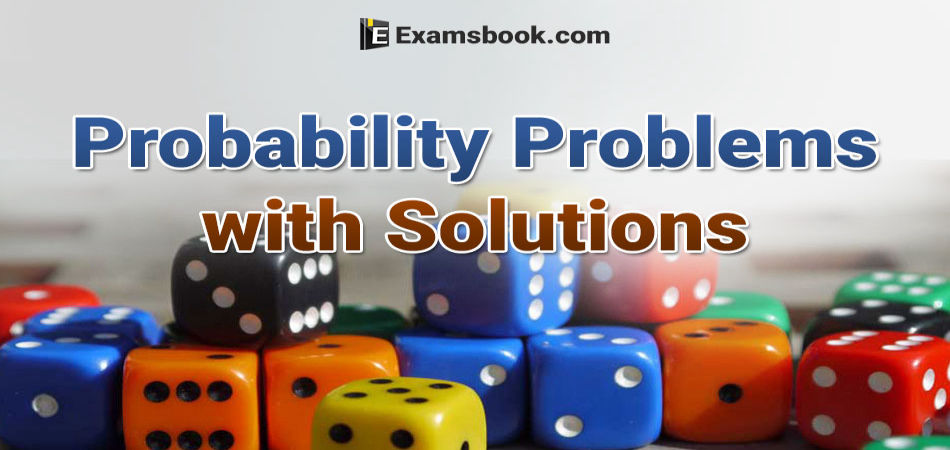• Save

# Probability problems with solutions for bank exams and SSC

Vikram Singh3 months ago 3.1K ViewsDo you think it is hard to solve probability problems in ssc or bank exams? Maybe it is hard to solve but here in this blog, I am sharing probability problems with solutions that will help you in your preparations.

With the help of these probability solutions with problems, you can learn how to solve probability questions and can improve your performance level. You can learn or practice formulas of probability with examples by one click.

## Probability Problems with Solutions for Competitive Exams

Q.1. In a single throw of a die, what is the probability of getting a number greater than 4 ?

Solution

When a die is thrown, we have S = {1,2,3,4,5,6}

Let E = event of getting a number greater than 4 = {5,6}

$$∴ P(E)={n(E)\over n(S)} = {2\over6}={1\over3}$$

Q.2. In a simultaneous throw of two dice, what is the probability of getting a total of 7 ?

Solution

We know that in a simultaneous throw of two dice, n (S) = (6×6)=36.

Let E = event of getting a total of 7 = {(1,6),(2,5),(3,4),(4,3),(5,2),(6,1)}.

$$∴ P(E)={n(E)\over n(S)} = {6\over36}={1\over6}$$

Q.3. What is the probability of getting a sum 9 from two throws of a dice ?

Solution

In two throws of a die, n(S) = (6×6)=36.

Let E = event of getting a sum = {(3,6),(4,5),(5,4),(6,3)}.

$$∴ P(E)={n(E)\over n(S)} = {4\over36}={1\over9}$$

Q.4. In a simultaneous throw of two dice, what is the probability of getting a doublet ?

Solution

In a simultaneous throw of two dice, n(S) = (6×6)=36.

Let E = event of getting a doublet = {(1,1),(2,2),(3,3)(4,4)(5,5)(6,6)}.

$$∴ P(E)={n(E)\over n(S)} = {6\over36}={1\over6}$$

Q.5. In a simultaneous throw of two dice, what is the probability of getting a total of 10 or 11 ?

Solution

In a simultaneous throw of two dice, We have n (S), = (6×6)=36.

Let E= event of getting a total of 10 or 11{(4,6),(5,5),(6,4)(5,6)(6,5)}

$$∴ P(E)={n(E)\over n(S)} = {5\over36}$$

Q.6. Tickets numbered 1 to 20 are mixed up and then a ticket is drawn at random. What is the probability that the ticket drawn bears a number which is a multiple of 3 ?

Solution

Here, S = {1,2,3,4,……..,19,20}.

Let E = event of getting a multiple of 3 = {3,6,9,12,15,18}.

$$∴ P(E)={n(E)\over n(S)} = {6\over20}={3\over 10}$$

Q.7. Tickets numbered 1 to 20 are mixed up and then a ticket is drawn at random. What is the probability that the ticket drawn has a number which is a multiple of 3 or 5 ?

Solution

Here, S = {1,2,3,4,………..,19,20}

Let E = event of getting a multiple of 3 or 5= {3,6,9,12,15,18,5,10,20}.

$$∴ P(E)={n(E)\over n(S)} = {9\over20}$$

Q.8. In a lottery, there are 10 prizes and 25 blanks. A lottery is drawn at random. What is the probability of getting a prize ?

Solution

P (getting a prize) $$= {10\over (10+25)} = {10\over 35} = {2\over7}$$

Q.9. One card is drawn at random from a pack of 52 cards. What is the probability that the card drawn is face card ?

Solution

Clearly, there are 52 cards, out of which there are 16 face cards.

∴ P (getting a face card) $$= {16\over 52} = {4\over13}$$

Q.10. A card is drawn from a pack of 52 cards. The probability of getting a queen of club or a king of heart is :

Solution

Here, n(S) = 52.

Let E = event of getting a queen of club or a king of heart.

Then, n(E) = 2. $$∴ P(E)={n(E)\over n(S)} = {2\over52}={1\over26}$$

Learn and practice these probability problems with solutions and you can ask me anything in the comment section if you have any doubts regarding probability problems and it's solutions. You can more practice with probability questions and answers by visit here.

All the best !!